Integer Programming

This full chapter is dedicated to integer programming.

The general linear programming model depends on the assumption of divisibility. In other words, the decision variables are allowed to take non-negative integer as well as fractional values. However, we quite often face situations where the planning models contain integer valued variables.

For instance, trucks in a fleet, generators in a powerhouse, pieces of equipment, investment alternatives and there are a myriad of other examples. In all such cases, an integer solution is desired, which can be easily obtained by rounding off the fractional values of the variables. But, rounding-off may result in sub-optimal or infeasible solutions. To overcome such difficulties, a different optimization model, which is referred to as integer programming has been developed.

Definitions: Integer Programming

Integer programming problem (or discrete programming problem) is a type of problem in which some, or all, of the variables are allowed to take only integral values.

The focus of this chapter is on solution techniques for integer programming models.

In this chapter, we drop the assumption of divisibility.

The mathematical model of the problem is as follows:

Optimize (maximize or minimize)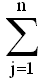cjxj

subject toaijxj ( ≤, =, ≥ ) bi; i = 1, 2, ....., m

xj ≥ 0; j = 1, 2, ....., n
xj integer valued; j = 1, 2, ....., p ≤ n

The discreteness stipulation distinguishes an integer from a linear programming problem.

If all the variables are restricted to take only integral values (i.e., p = n), the model is called a pure integer programming problem. To the contrary, if some variables are restricted to take only integer values, and the remaining are free to take any non-negative values, then it is called a mixed integer programming problem. When the decision variables are required to take value of either zero or one, it is called zero-one programming.

The mathematical model of zero-one programming is as follows:

Optimize (maximize or minimize)cjxj

subject toaijxj ( ≤, =, ≥ ) bi; i = 1, 2, ....., m

xj = 0 or 1; j = 1, 2, ....., n

•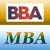MBA & BBA
•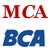BCA & MCA
•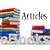Ebooks & Articles
•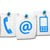Contact us